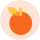# FILTER POSTS

•### Here's how to be a Super Saver!

In this article, we'll explain to students in Key Stage 1 the importance of understanding money, and…

•### Find the difference using addition and subtraction

In year 2, students should be able to add and subtract numbers using concrete objects, pictorial rep…

•### Multiplication - Let's simplify that for you!

In year 2, students should be able to solve problems involving multiplication and division, using ar…

•### Want to simplify subtraction?

In Year 2, pupils should be able to add and subtract numbers using concrete objects, pictorial repre…

••